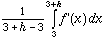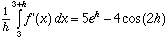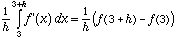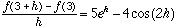Date: Mon, 19 Apr 1999 20:41:37 -0600 (CST) Sender:Tammy Subject: calculus Name: Tammy Who is asking: Student Level: Secondary Question: suppose that the average rate of change of a function f over the interval from x=3 to x=3+h is given by 5e^h-4cos(2h). what is f'(3)? I would appreciate any help with this question. Hi Tammy The rate of change of f is f' so the average rate of change of f from 3 to 3+h isThusSince f is an antiderivative of f',ThusNow take the limit of both sides as h approaches 0. Cheers Harley

Go to Math Central